Next: Tensors Up: Relativity and electromagnetism Previous: The Lorentz transformation

## Transformation of velocities

Consider two frames,and, in the standard configuration. Letbe the velocity of a particle in. What is the particle's velocity in? The components ofare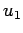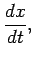(1354)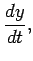(1355)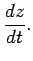(1356)

Similarly, the components of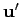are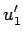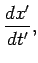(1357)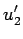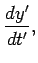(1358)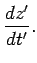(1359)

Now we can write Eqs. (1346)-(1349) in the form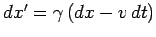,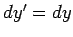,, and, where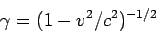(1360)

is the well-known Lorentz factor. If we substitute these differentials into Eqs. (1354)-(1356), and make use of Eqs. (1357)-(1359), we obtain the transformation rules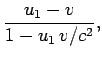(1361)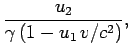(1362)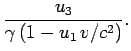(1363)

As in the transformation of coordinates, we can obtain the inverse transform by interchanging primed and unprimed symbols, and replacingwith. Thus,(1364)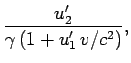(1365)(1366)

Equations (1364)-(1366) can be regarded as giving the resultant,, of two velocities,and, and are therefore usually referred to as the relativistic velocity addition formulae. The following relation between the magnitudes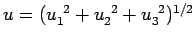andof the velocities is easily demonstrated: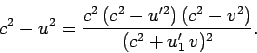(1367)

If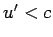andthen the right-hand side is positive, implying that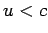. In other words, the resultant of two subluminal velocities is another subluminal velocity. It is evident that a particle can never attain the velocity of light relative to a given inertial frame, no matter how many subluminal velocity increments it is given. It follows that no inertial frame can ever appear to propagate with a superluminal velocity with respect to any other inertial frame (since we can track a given inertial frame using a particle which remains at rest at the origin of that frame).

According to Eq. (1367), if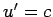then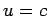, no matter what valuetakes: i.e., the velocity of light is invariant between different inertial frames. Note that the Lorentz transform only allows one such invariant velocity [i.e., the velocity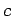which appears in Eqs. (1346)-(1349)]. Einstein's relativity principle tells us that any disturbance which propagates through a vacuum must appear to propagate at the same velocity in all inertial frames. It is now evident that all such disturbances must propagate at the velocity. It follows immediately that all electromagnetic waves must propagate through the vacuum with this velocity, irrespective of their wavelength. In other words, it is impossible for there to be any dispersion of electromagnetic waves propagating through a vacuum. Furthermore, gravity waves must also propagate with the velocity.

The Lorentz transformation implies that the velocities of propagation of all physical effects are limited byin deterministic physics. Consider a general process by which an event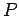causes an event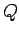at a velocityin some frame. In other words, information about the eventappears to propagate to the eventwith a superluminal velocity. Let us choose coordinates such that these two events occur on the-axis with (finite) time and distance separations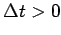and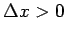, respectively. The time separation in some other inertial frameis given by [see Eq. (1349)](1368)

Thus, for sufficiently largewe obtain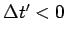: i.e., there exist inertial frames in which cause and effect appear to be reversed. Of course, this is impossible in deterministic physics. It follows, therefore, that information can never appear to propagate with a superluminal velocity in any inertial frame, otherwise causality would be violated.Next: Tensors Up: Relativity and electromagnetism Previous: The Lorentz transformation
Richard Fitzpatrick 2006-02-02